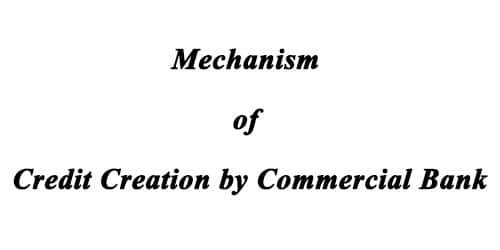# Mechanism of Credit Creation by Commercial Bank

Mechanism of Credit Creation by Commercial Bank

A commercial bank is a financial bank which takes money from the people as a deposit and gives money to the other people as a loan. This bank gives minimum interest to depositors and demand maximum interest from the borrower.

Let explain the actual process of credit creation. We know that the ability of banks to create credit depends on the fact that banks need only a small percentage of cash to deposits. If banks kept 100 percent cash against deposits, there would be no credit creation. Modern banks do not keep 100 percent cash reserves.

They are legally required to keep a fixed percentage of their deposits in cash, say 10, 15 or 20 percent. They lend and/or invest the remaining amount which is called excess reserves. A bank can lend equally to its excess reserves. But the entire banking system can lend and create credit (or deposits) up to a multiple of its original excess reserves. The deposit multiplier depends upon the required reserve which is the basis of credit creation. Symbolically, the required reserve ratio:

RRr = RR/D

Or, RR = RRr x D

Where RR is the required cash reserves with banks, RRr is the required reserve ratio and D is the demand deposits of banks. To show that D depends on RR and RRr, divide both sides of the above equation by RRr;

RR/RRr = RRr x D/RRr

Or, RR/RRr = D

Or, 1/RRr = D/RR

Or, D = 1/RRr x RR

Where 1/RRr, the reciprocal of the percentage reserve ratio, is called the deposit (or credit) expansion; the limits of the deposit expansion of a bank. The maximum amount of demand deposits which the banking system can support with any given amount of RR is by applying the multiplier to RR. Taking the initial change in the volume of deposits (DD) and in cash reserves (DRR), it follows from any given percentage of RRr that,

To understand it, suppose the RRr for the banks is fixed at 10 percent and the initial change in cash reserves is Rs 1000. By applying the above formula, the maximum increase in demand deposits will be –

AD = 1000 x 1/0.10 = Rs. 10000.

This is the extent to which the banking system can create credit. The above equation can also be expressed as follows: DD = RR [1 + (l-RRr) + (Y-RRr)2 + … + (l-RRr)n]

The sum of the geometric progression within brackets gives:

1/1 – (1 – RRr) = l/RRr